## Solution to 1986 Problem 25

 The Lorentz force law is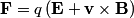\begin{align*}\mathbf{F} = q \left(\mathbf{E} + \mathbf{v} \times \mathbf{B} \right)\end{align*}Initially,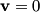$\mathbf{v} = 0$, so the second term inside the parenthesis is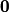$\mathbf{0}$. So, at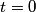$t = 0$, the particle experiences a force in the direction of the electric field, which gives the particle a velocity in the direction of the electric field. Since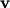$\mathbf{v}$ and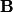$\mathbf{B}$ are parallel, the second term inside the parenthesis is still$\mathbf{0}$. So, the particle will continue to move in a straight line and not experience any magnetic force. Therefore, answer (E) is correct.